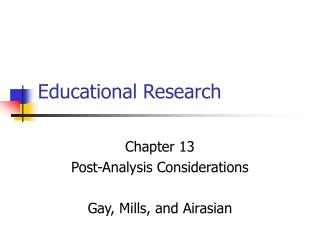Download PresentationEducational Research

# Educational Research - PowerPoint PPT PresentationDownload Presentation## Educational Research

- - - - - - - - - - - - - - - - - - - - - - - - - - - E N D - - - - - - - - - - - - - - - - - - - - - - - - - - -
##### Presentation Transcript

1. Educational Research Chapter 13 Post-Analysis Considerations Gay, Mills, and Airasian

2. Topics Discussed in this Chapter • Verification and storage of data • Checking, organizing, and evaluating data • Interpretation of results • Hypothesized and unhypothesized results • Statistical issues • Methodological issues • Statistical and practical significance • Replication

3. Verifying and Storing Data • Three issues • Checking data • Organizing data • Evaluating the research conclusions • Checking data for accuracy • Check the original data • Check the coded data against the initial uncoded data • Electronic data • Print the data sets and check them • Check the output carefully Objective 1.1

4. Verifying and Storing Data • Organizing data • Label the data • Dates • Important details related to the data itself and the study • Store the data • Multiple copies • Multiple sites Objective 1.2

5. Verifying and Storing Data • Evaluate the research conclusion(s) • Check the accuracy of the computations • Check the reasonableness of the results Objective 1.3

6. Interpreting the Results • Interpretations are made in light of … • the purpose of the study, • the original research hypotheses, and • the findings of other related studies. • Hypothesized results • Results are interpreted relative to their support or lack of support of the research hypotheses Objectives 2.1 & 3.1

7. Interpreting Results • Hypothesized results (continued) • Supported hypotheses • Probabilistic nature of the decision • Easily interpreted given the control and manipulation used in the research design • Non-supported hypotheses • Probabilistic nature of the decision • Possible importance of knowing what doesn’t work • Potential methodological problems Objectives 2.1 & 3.1

8. Interpreting Results • Unhypothesized results • Results that appear during the study • Must be interpreted with great care • Researchers base their studies on ideas related to what will happen, not what is happening • Possible importance to and influence on later studies Objective 4.1 & 4.2

9. Interpreting Results • Statistical influences • The sample must be representative of the population • The assumptions of the specific statistical tests of significance must be met Objective 5.1

10. Interpreting Results • Three methodological influences • Measurement error – large amounts of error hamper the ability to find statistically significant results • Statistical power • The probability of avoiding a Type II error (i.e., the probability of rejecting the null hypothesis when it should be rejected) Objectives 5.2 & 6.1

11. Interpreting Results • Three methodological factors (cont.) • Statistical power (cont.) • Three influential factors • Sample size – the larger the sample the greater the power • Significance levels and directionality of the test • Increasing alpha (e.g., .01, .05, .10) increases power • One-tailed tests are more powerful Objectives 5.2 & 6.2

12. Interpreting Results • Three methodological factors (cont.) • Statistical power (cont.) • Effect size • Larger effect sizes increase power • Effect sizes can be estimated from prior studies, estimated by minimal levels below which the results are deemed unimportant, and agreed upon levels of small (.20), moderate (.50), and large (.80). • A priori and post-hoc considerations of power Objectives 5.2 & 6.2

13. Interpreting Results • Three methodological factors (cont.) • Multiple comparisons – the more statistical tests of significance the greater the likelihood of falsely rejecting the null hypothesis Objective 5.2

14. Interpreting Results • Statistical and practical significance • Statistical significance relates to the tests of significance being used • Practical significance relates to the usefulness of the results • Statistical and practical significance are not necessarily the same • Statistical significance depends on sample size • Studies with large samples can be significant but of little value Objective 5.3

15. Interpreting Results • Statistical and practical significance (cont.) • Precision and accuracy • Precision relates to how narrowly an estimate is specified • Accuracy relates to how close an estimate is to the true value Objective 5.4

16. Interpreting Results • Replication • Replication involves repeating the study • Different subjects • Different context • Different times • Extremely important when… • an unusual or new result is found, or • the results have high levels of practical significance. Objective 7.1